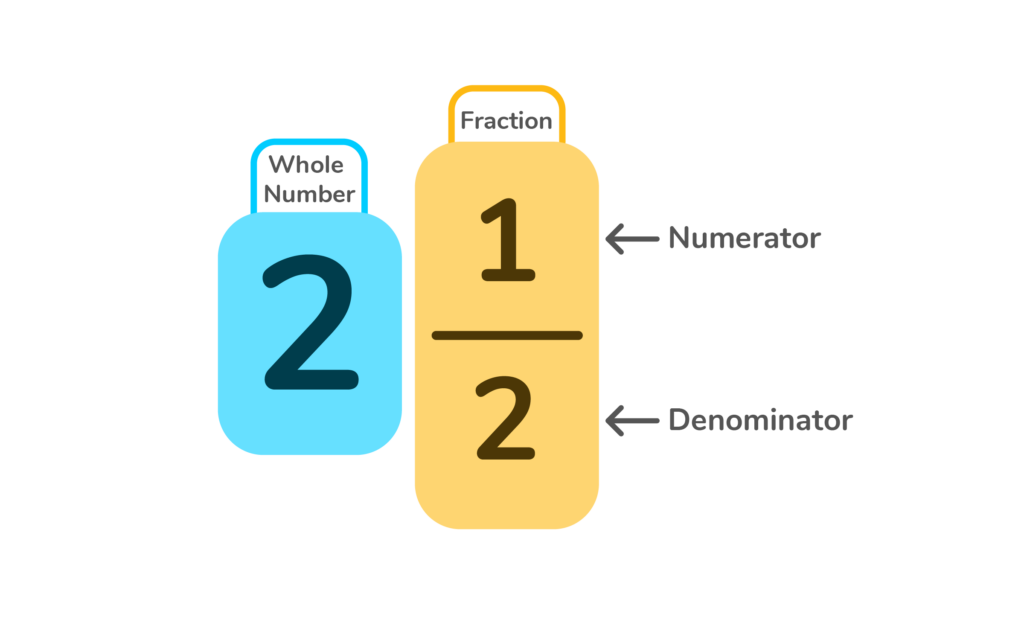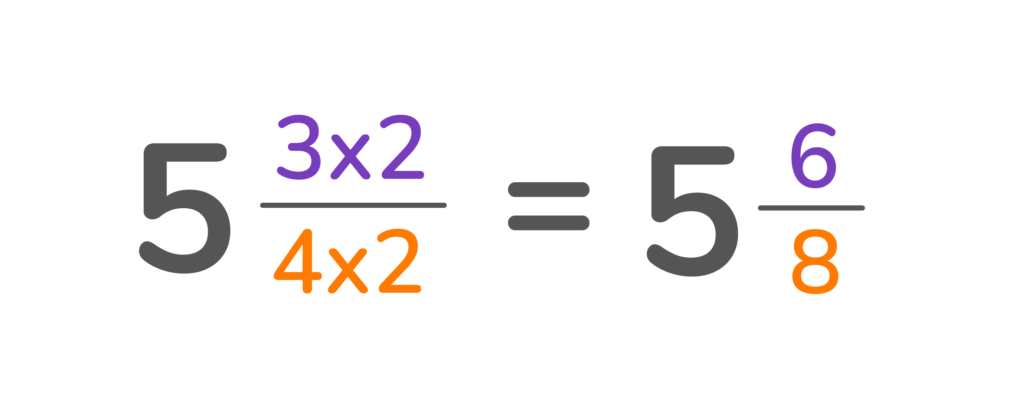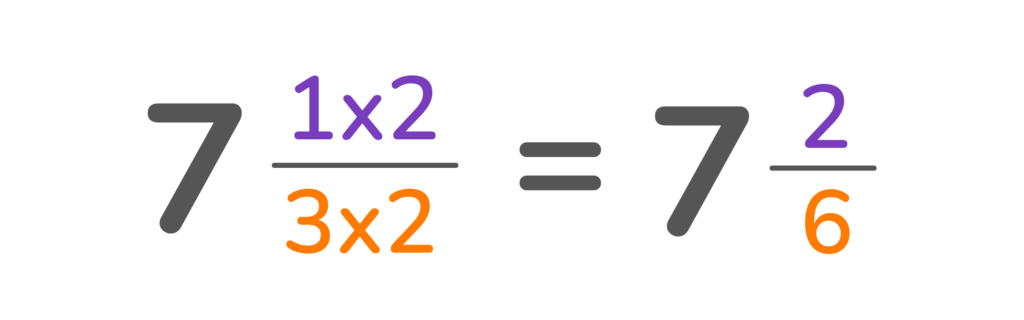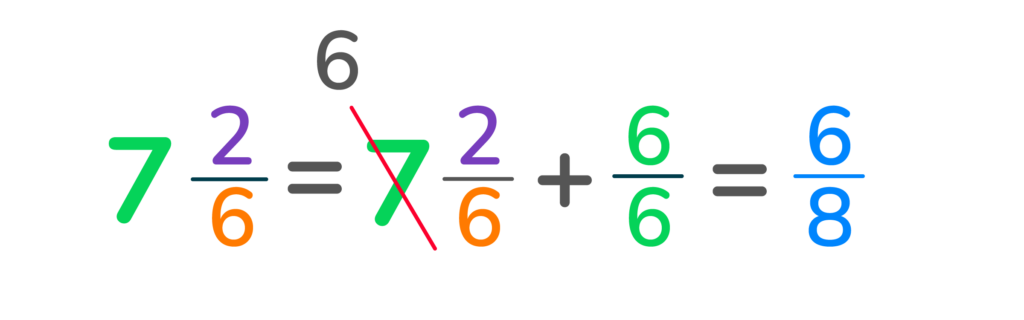# How to Subtract Mixed Numbers

This comprehensive guide provides step-by-step instructions to solve different types of mixed number subtraction problems.Author
Katie WickliffPublished
October 19, 2023# How to Subtract Mixed Numbers

This comprehensive guide provides step-by-step instructions to solve different types of mixed number subtraction problems.Author
Katie WickliffPublished
Oct 19, 2023# How to Subtract Mixed Numbers

This comprehensive guide provides step-by-step instructions to solve different types of mixed number subtraction problems.Author
Katie WickliffPublished
Oct 19, 2023Key takeaways

• Mixed numbers consist of a whole number and a fractional part, such as 4 ⅓
• Finding the least common denominator is a key part of subtracting mixed numbers with unlike denominators
• Some mixed number subtraction problems require students to regroup, or “borrow”

When your child is learning how to subtract mixed numbers, remembering the steps to the process may seem a little challenging at first. However, with quality instruction and a solid practice routine, your student will soon be able to subtract several types of mixed numbers. This article provides a complete overview of how to subtract mixed numbers with the same and different denominators.

## What are mixed numbers?Before subtracting mixed numbers, students need to understand that a mixed number is comprised of a whole number and part of a number, which is also called a fraction. Students should be able to identify each component and recognize both the numerator and the denominator of the fraction, like this:## Subtracting mixed numbers step by stepWhen learning to subtract mixed numbers, reviewing the process one step at a time can help students recognize each part of the mixed number and determine how to solve for that particular problem.

### Subtracting mixed numbers with like denominatorsTo subtract mixed numbers with like denominators, follow the steps below.

Let’s use 6 ⅔ – 2 ⅓ as an example.

First, subtract the whole numbers.

6-2=4

Next, subtract the fractions.

⅔-⅓=⅓

Finally, write the mixed number answer.

4 ⅓

### Subtracting mixed numbers with unlike denominatorsTo subtract mixed numbers with unlike–or unequal–denominators, let’s use the example problem of 5 ¾- 2 ⅛

First, we need to look at the fractions and find the least common denominator.

¾ and ⅛ have a least common denominator of 8.

What is the least common denominator?

The least common denominator, also known as the lowest common denominator, is the smallest number the denominators can be divided into.

Example:

The denominator in ¾ is 4.

4 can be divided into 1,2, and 4.

The denominator in ⅛ is 8. 8 can be divided into 1,2,4,8.

The smallest number the denominators both have in common is 4.

4 is the least common denominator.

Next, we need to rename the fraction using the least common denominator.

To do this, we multiply both the bottom and top of the fraction.

5 (3×2/4×2) = 5 6/8Now that the two fractions have equal denominators, we subtract the mixed numbers using the process above.

5 6/8- 2 ⅛=

5-2= 3

6/8- ⅛= ⅝

3 ⅝

### Subtracting mixed numbers with regroupingLet’s look at the example problem of 7 ⅓- 4 ⅚

First, we need to find the least common denominator.

⅓ and ⅚ have a least common denominator of 6.

Next, we need to rename the fraction using the least common denominator.

To do this, we multiply both the bottom and top of the fraction.Now we can rewrite the problem as:

7 2/6- 4 ⅚

Even though our mixed numbers now have the same denominator, we can’t subtract 2/6 from ⅚ because we’d get a negative number.

Similar to a whole number subtraction problem, we need to borrow one whole from the place to the left and add it to the right — but in fractional form.Now, we have 7 8/6 and can easily subtract the like denominators.

7 8/6- 4 ⅚ = 3 3/6 or 3 ½

## Practice problems

3 ⅚- 2 ⅙=?

3-2 = 1

⅚-⅙= 4/6

6 8/9-5 ⅔=?

Find the least common multiple of 3 and 9, which is 9.

5 (2×3/3×3)= 5 6/9

6 8/9- 5 6/9=

9 ⅞- 5 ⅛=?

9-5= 4

⅞- ⅛= 6/8

2 12/9- 1 5/9= 1 7/9

Find the least common multiple of 3 and 6, which is 6.

4 (1×2/3×2)= 4 2/6

4 2/6- 1 ⅙=

## FAQs about subtracting mixed numbersTo subtract mixed numbers, you must first find the least common denominator of each. Then you can subtract or regroup in order to subtract.

In order to subtract a whole number from a mixed number, you need to first convert the whole number into a fraction. Then, you need to change the mixed number into an improper fraction. Finally, find the common denominator of the two fractions in order to subtract.Lesson credits

Katie Wickliff

Katie holds a master’s degree in Education from the University of Colorado and a bachelor’s degree in both Journalism and English from The University of Iowa. She has over 15 years of education experience as a K-12 classroom teacher and Orton-Gillingham certified tutor. Most importantly, Katie is the mother of two elementary students, ages 8 and 11. She is passionate about math education and firmly believes that the right tools and support will help every student reach their full potential.

Katie Wickliff

Katie holds a master’s degree in Education from the University of Colorado and a bachelor’s degree in both Journalism and English from The University of Iowa. She has over 15 years of education experience as a K-12 classroom teacher and Orton-Gillingham certified tutor. Most importantly, Katie is the mother of two elementary students, ages 8 and 11. She is passionate about math education and firmly believes that the right tools and support will help every student reach their full potential.# Are you a parent, teacher or student?

Are you a parent or teacher?

## Hi there!

Book a chat with our team